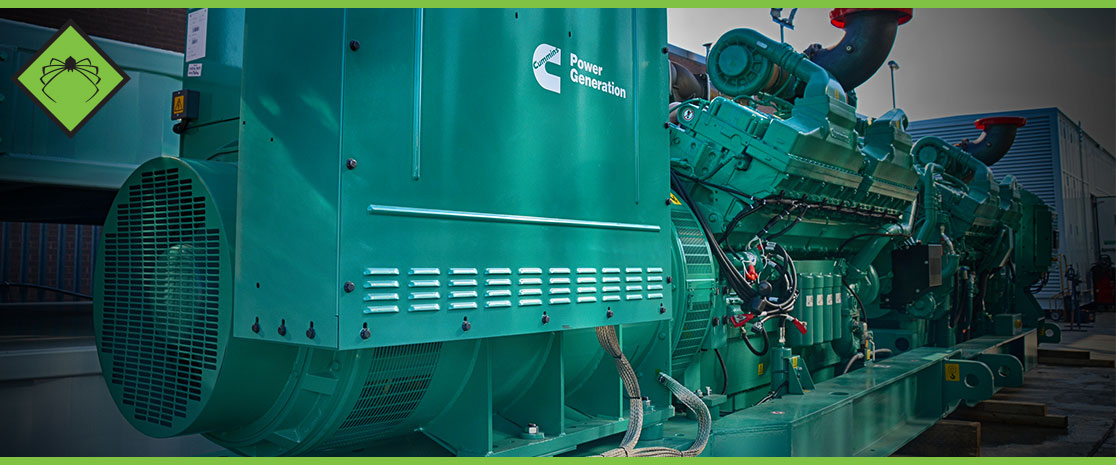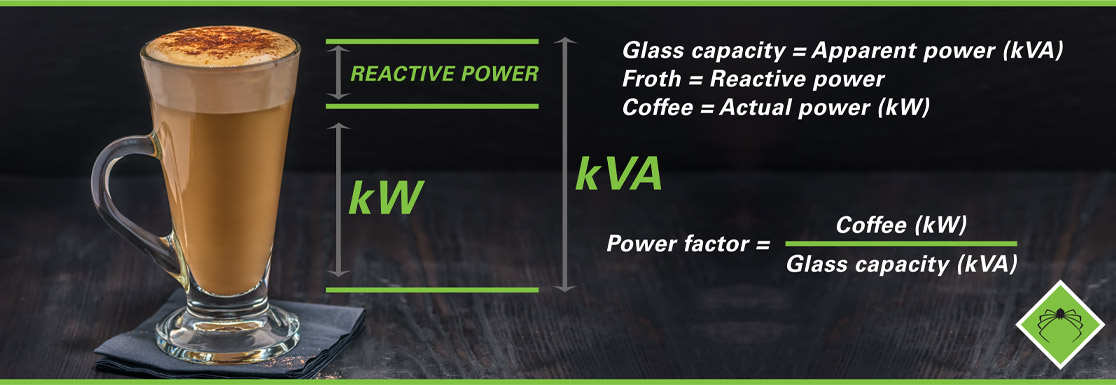# What is kVA and How is it Calculated?

Power ratings are expressed in different forms such as watts and kilowatts, amperes or amps, volts, and also in kVA, But what exactly is kVA?

## The kVA Power Rating

Outside the generator industry, the term kilovolt-amperes (kVA) is not well known. A kilowatt (kW) is a much more common term and is how electrical items in your home are rated, you may even notice it quantified on your electricity bill so it is much more relatable, but what exactly is kVA, why is it used and how is it calculated?

### kVA Calculator

Use our handy calculator below to quickly convert kW to kVA, or to convert kVA to kW:

#### Actual Power

Therefore we can refer to kW (kilowatts) as actual power, it is the amount of power that is converted into an output.

#### Apparent Power

On the other hand kVA (kilovolt-amps) is a measure of apparent power: it describes the total amount of power being used by a system, for example in a 100% efficient system kW would equal kVA exactly. However in reality electrical systems are not 100% efficient and so not all of the system's apparent power is being used for useful work output.

Fundamentally, 1 kVA is equal to 1,000 volt amps. Whereas volts is a measurement of electrical pressure, and amps is a measure of electrical current. A term called apparent power (the absolute value of complex power) is equal to the product of the volts and amps.

#### Power Factor

Most 3 phase generators have a power factor of 0.8. When you know this it is easy to convert kVA to kW becasue you know the efficiency level of the electrical system in question. Electrical efficiency is usually expressed as a power factor inbetween 0 & 1, therefore the closer the power factor is to 1, then the more efficiently the kVA is being converted into actual kilowatts.

##### The kVA to kW / kW to kVA Formulas

kVA to kW Apparent power (kVA) x power factor (pf) = actual power (kW) e.g. 100 kVA x 0.8 = 80 kW

kW to kVA Actual power (kW) / power factor (pf) = apparent power (kVA) e.g. 80 kW / 0.8 = 100 kVA

###### How Does kVA Work?

The Coffee Analogy

Use our coffee analogy to gain a better understanding of the relationship between kW, kVA & power factor:• Glass capacity = Apparent power (kVA)
• Froth = Reactive power
• Coffee = Actual power (kW)
• Power factor = Coffee (kW) / Glass capacity (kVA)